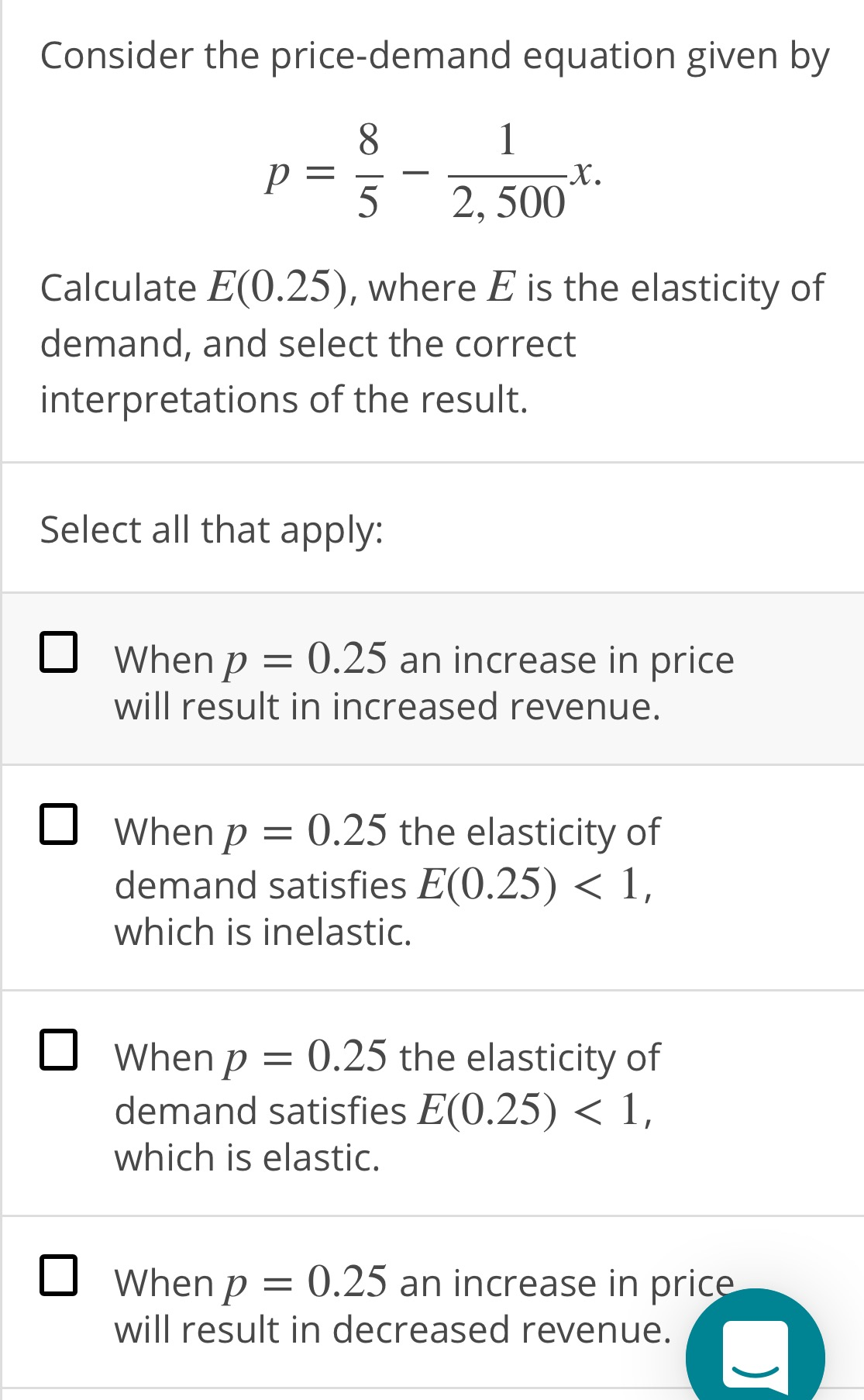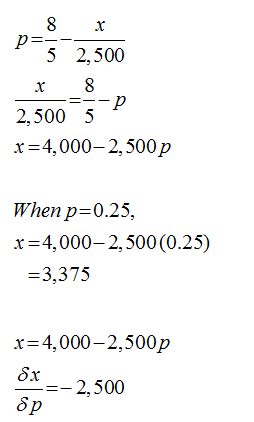# Consider the price-demand equation given by8.52, 500Calculate E(0.25), where E is the elasticity ofdemand, and select the correctinterpretations of the result.Select all that apply:O When p = 0.25 an increase in pricewill result in increased revenue.O When p = 0.25 the elasticity ofdemand satisfies E(0.25) < 1,which is inelastic.When p = 0.25 the elasticity ofdemand satisfies E(0.25) < 1,which is elastic.O When p =will result in decreased revenue.0.25 an increase in price

Question
1 viewshelp_outlineImage TranscriptioncloseConsider the price-demand equation given by 8. 5 2, 500 Calculate E(0.25), where E is the elasticity of demand, and select the correct interpretations of the result. Select all that apply: O When p = 0.25 an increase in price will result in increased revenue. O When p = 0.25 the elasticity of demand satisfies E(0.25) < 1, which is inelastic. When p = 0.25 the elasticity of demand satisfies E(0.25) < 1, which is elastic. O When p = will result in decreased revenue. 0.25 an increase in price fullscreen
check_circle

Step 1

The price demand equation is:...

### Want to see the full answer?

See Solution

#### Want to see this answer and more?

Solutions are written by subject experts who are available 24/7. Questions are typically answered within 1 hour.*

See Solution
*Response times may vary by subject and question.
Tagged in